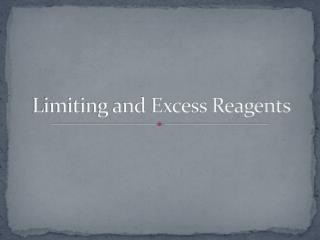DownloadDownload PresentationLimiting and Excess Reagents

# Limiting and Excess Reagents

Download Presentation## Limiting and Excess Reagents

- - - - - - - - - - - - - - - - - - - - - - - - - - - E N D - - - - - - - - - - - - - - - - - - - - - - - - - - -
##### Presentation Transcript

1. Limiting and Excess Reagents

2. In any chemical reaction, it is easy to run out of one or another reactant – which has an impact on the amount of products that can result from a reaction • To solve these problems you must identify which of the reactants is going to run out first. • This is the “limiting reagent” • The other is the “excess reagent” • Example: LEGO – If you had three yellow blocks and four red blocks, you could only make three yellow/red combinations – because there is not enough yellow blocks to make four. The yellow block is the limiting reagent and the red block is the excess reagent + =

3. Identifying Limiting and Excess Reagents • WHY DO WE CARE?? • It is often desirable to know how much excess reagent is required to ensure that a reaction goes to completion. • When you know the quantity of more than one reagent, you may need to know which one will limit the reaction.

4. 1) If 10.0 mol of methane and 10.0 mol of oxygen react, which is the limiting reagent. 10.0 mol 10.0 mol Use one of the amounts of reactants to test which reactant is the limiting reagent CH4 = 10.0 mol CH4 2 O2 x mol O2 x = 20 .0 mol CH4(g) + 2 O2(g)  CO2(g) + 2 H2O(g) If we had used the given amount of O2 to calculate the amount of CH4 required, we would find that 5.00 mol of methane would be required to react with 10.0 mol of oxygen 20.0 mol of oxygen would be required to react with 10.0 mol of methane Since 20.0 mol of oxygen is required to react with 10.0 mol of methane, but only 10.0 mol is available, oxygen is the limiting reagent. How much methane would be left? 10.0 mol – 5.00 mol = 5.00 mol

5. a) If 10.0g of copper is placed in solution of 20.0g of silver nitrate, which reagent will be the limiting reagent? • All reactants must be converted to moles, then using the mole ratio, determine which reactant will run out first. Cu(s) + 2 AgNO3(aq) 2 Ag(s) + Cu(NO3)2(aq) 10.0g 20.0g 63.55 g/mol 169.88g/mol n Cu(s): 10.0g = 0.157 mol 63.55 g/mol n AgNO3(aq): 20.0g = 0.118 mol 169.88 g/mol That much silver nitrate is not available so copper is not the limiting reagent More copper than that is available so silver nitrate is the limiting reagent x = 0.314 mol of AgNO3 are needed to react with 0.157 mol of Cu. We only have 0.118 mol of AgNO3, therefore AgNO3 is the LIMITING REAGENT

6. b) From the previous example, where 10.0 g of copper reacts with 20.0 g of silver nitrate, what mass of copper will be in excess? (leftover when the reaction is complete) Cu(s) + 2 AgNO3(aq) 2 Ag(s) + Cu(NO3)2(aq) 0.157 mol 0.118 mol 0.157 mol – 0.059 = 0.098 mol of Cu will be left over m = 0.098 mol (63.55 g/mol) = 6.2g Since AgNO3 is the limiting reactant, use it’s number of moles to determine how much product (Ag) you can get. x = 0.118 mol of Ag can be produced m = n MM = 0.118 mol (107.87 g/mol) = 12.7 g XS LR c) What mass of silver will be produced?

7. Putting it all together… • In an experiment, 26.8g of iron (III) chloride in solution is combined with 21.5g of sodium hydroxide. Which reactant is in excess, and by how much? What mass of precipitate will be obtained? FeCl3(aq) + 3NaOH(aq)  Fe(OH)3(s) + 3NaCl(aq) 26.8g 21.5g m = ? 162.20g/mol 40.00g/mol 106.88g/mol nFeCl3 = 26.8g = 0.165 mol 162.20g/mol nNaOH = 21.5 g = 0.538 mol 40.0g/mol Test one of the reactants to find out which one is the limiting reactant. Therefore, 0.495 mol of NaOH are Needed to react with 0.165 mol of FeCl3. We have 0.538 mol of NaOH, Therefore NaOH is the XS reagent and FeCl3 is the LIMITING REAGENT.

8. Which reactant is in excess, and by how much? NaOH is in excess. Subtract the amount of NaOH given from the amount needed to react with the number of moles of limiting reactant. XS NaOH = 0.538mol NaOH – 0.496 mol NaOH = 0.042 mol NaOH Find the mass of xs NaOH m=n MM = 0.042 mol x 40.00 g/mol = 1.7 g NaOH (excess) What mass of precipitate will be obtained? Compare the number of moles of LR to the amount of precipitate you can form from the balanced equation. Find the mass of precipitate (product) m = n MM = 0.165 mol x 106.88 g/mol = 17.7 g of Fe(OH)3(s)

9. Limiting and Excess Reagents Summary • Identify the limiting reagent by choosing either reagent amount, and use the mole ratio to compare the required amount with the amount actually present. • The quantity in excess is the difference between the amount of excess reagent present and the amount required for complete reaction.

10. Homework • Read 7.3 and 7.4 • Pg 330 # 2,3,6,7 • Pg 335 # 1,2,3